# Section Formula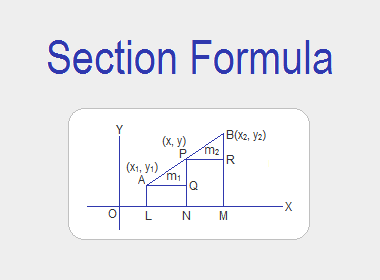Section formula is a formula to calculate the coordinates of any point P(x, y) which divides the line joining the points A(x1, y1) and B(x2, y2) in the ratio of m1:m2 internally or externally. Here are those formulas:

**********************

10 Math Problems officially announces the release of Quick Math Solver, an android APP on the Google Play Store for students around the world.**********************

(i)  If P(x, y) divides AB internally in the ratio of m1:m2, then the section formula for internal division is given by,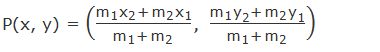(ii) If P(x, y) divides AB externally in the ratio of m1:m2, then the section formula for external division is given by,## Derivation of Section Formula

### Internal Division

Let A(x1, y1) and B(x2, y2) be given two points. Let the point P(x, y) divides the line joining AB internally in the ratio of m1:m2. Then, AP:PB = m1:m2

Draw perpendiculars AL, PN and BM from A, P and B respectively to the x-axis. Then, OL = x1, ON = x, OM = x2, AL = y1, PN = y and BM = y2. Again draw perpendicular AQ and PR from A and P to the line segments PN and BM respectively.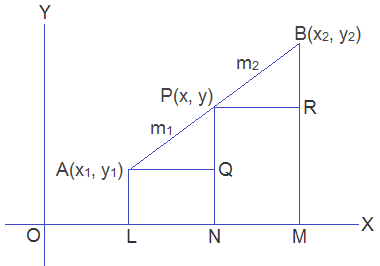Then,

AQ = LN = ON – OL = x – x1

PR = NM = OM – ON = x2 – x

PQ = PN – QN = PN – AL = y – y1

BR = BM – RM = BM – PN = y2 – y

Now, from the figure, In ΔPQA and ΔBRP,

i.     PQA = BRP ---> both right angles

ii.    QAP = RPB ---> corresponding angles

iii.  APQ = PBR ---> corresponding angles

DPQA ~ DBRP ------> by AAA axiom### External Division

Let A(x1, y1) and B(x2, y2) be given two points. Let the point P(x, y) divides the line joining AB externally in the ratio of m1:m2. Then, AP:PB = m1:m2

Draw perpendiculars AL, BM and PN from A, B and P respectively to the x-axis. Then, OL = x1, OM = x2, ON = x, AL = y1, BM = y2 and PN = y. Again draw perpendiculars AQ from A to PN  and PR from P to BM produced.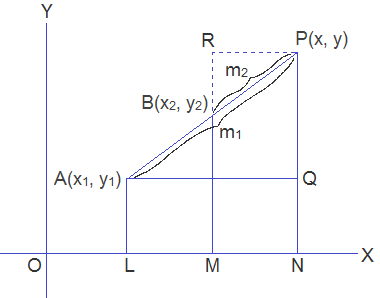Then,

AQ = LN = ON – OL = x – x1

PR = NM = ON – OM = x – x2

PQ = PN – QN = PN – AL = y – y1

BR = RM – BM = PN – BM = y – y2

In ΔPQA and ΔBPR,

i.     PQA = BRP ---> both right angles

ii.    QAP = RPB ---> corresponding angles

iii.  APQ = PBR ---> corresponding angles

DPQA ~ DBRP ------> by AAA axiomNote: The coordinates in the external division are obtained from those of the internal division by writing – m2 for m2.

### Mid-point Formula

If P(x, y) is the mid-point of the line joining A(x1, y1) and B(x2, y2), then the point P(x, y) divides the line AB internally in the ratio of 1:1. Therefore, by applying the section formula for internal division,### K-formula

If P(x, y) divides the line joining the points A(x1, y1) and B(x2, y2) in the ratio of k:1, then,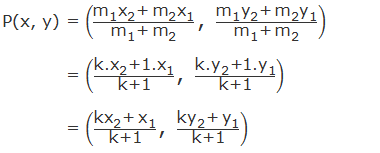It is a section formula in terms of k, which is called K-formula.

It is convenient to let the ratio as k:1 while solving the problems to find the ratio when the points are given. In such situations, we use the K-formula. Look at the worked-out examples below.

### Centroid Formula

Medians of a triangle intersect at a common point which is called the centroid of a triangle. If A(x1, y1), B(x2, y2) and C(x3, y3) are the vertices of a triangle ABC and G is the median, then the coordinates of centroid G is given by the following formula,#### Proof of Centroid Formula:

Let A(x1, y1), B(x2, y2) and C(x3, y3) are the vertices of a triangle ABC. D, E and F are the mid-points of BC, CA and AB respectively. And, medians AD, BE and CF intersect at point G.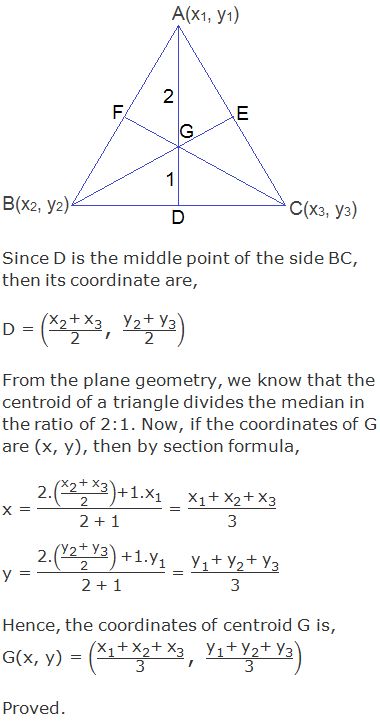## Worked Out Examples

Example 1: Find the co-ordinates of the point which divides the line joining the points (-3, -2) and (2, 8) in the ratio of 3:2 internally.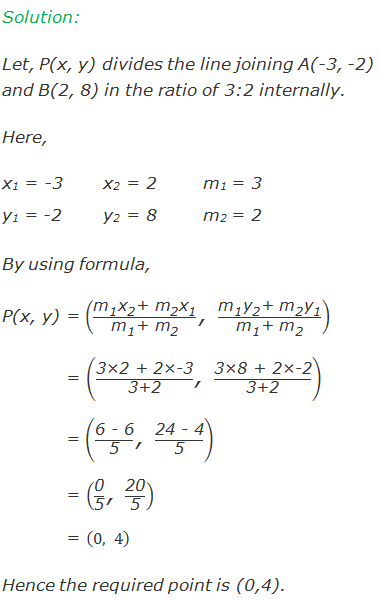Example 2: What ratio is the line joining (-3, 4) and (2, -6) divided by the point (-1, 0)?Example 3: If the points A (6, 4), B (a, 2), and C (1, -1) are three points on the same straight line, find the ratio in which the point  B divides the line segment AC and also find the value of a.Example 4: Find the coordinates of the points of trisection of the line joining the points M (7, -3) and N (1, 3).

Solution:

Points of trisection of a line segment joining two points are those points which divides the given line segment into three equal parts.

Let, P(a, b) and Q(c, d) are the points of trisection of the line segment joining the points M(7, -3) and N(1, 3). So P(a, b) divides MN in the ratio of 1:2, and hence,Hence, the required points of trisection are P(5, -1) and Q(3, 1).

Example 5: Find the centroid of the triangle with vertices A(-2, 4), B(11, 15) and C(3, -1).

Solution:

Let G(x, y) be the centroid of the triangle ABC with vertices A(-2, 4), B(11, 15), and C(3, -1). By using the formula of centroid,Hence, the centroid of the triangle is G(4, 6).

If you have any questions or problems regarding the Section Formula, you can ask here, in the comment section below.